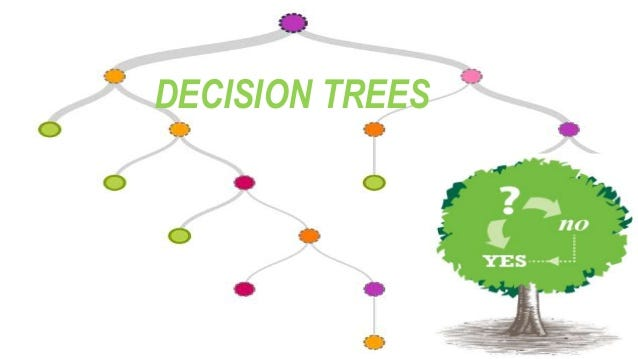# This is Tree! Decision Tree!!

Original article was published on Artificial Intelligence on MediumToday we will be focusing more on Decision Tee Regression and not on classification

# Decision Tee Regression

Decision Tee Regression consists of supervised machine learning algorithms to predict the output target (A target output is the true output or labels on a given dataset.) feature by an optimal recursive binary splitting of output target and input predictor features data into an incrementally smaller node.

The Top node is known as the Root Node, internal nodes are known as Decision Nodes, and finally, the terminal nodes are known as Leaf Nodes.

An Optimal Recursive Binary Splitting or just Recursive Binary Splitting is a numerical procedure where all the values are lined up and different split points are tried and tested using a cost function. The split with the best cost (lowest cost because we minimize cost) is selected.

A cost function is a measure of how wrong the model is in terms of its ability to estimate the relationship between x and y. This is typically expressed as a difference or distance between the predicted value and the actual value.